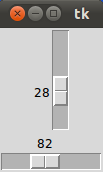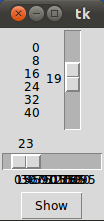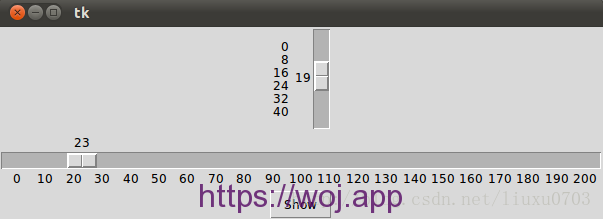A-A+

# [Tkinter Gui 教程09] Scale 控件

2019年12月20日 11:23 阅读 214 views 次

## 简介

Tkinter 中的 Scale 控件是一种可供用户通过拖动指示器改变变量值的控件. 这种控件可以水平放置, 也可以竖直放置.

## 简单例子

``````from Tkinter import *

master = Tk()
w = Scale(master, from_=0, to=42)
w.pack()
w = Scale(master, from_=0, to=200, orient=HORIZONTAL)
w.pack()

mainloop()``````## 获取 Scale 控件的值

``````from Tkinter import *

def show_values():
print (w1.get(), w2.get())

master = Tk()
w1 = Scale(master, from_=0, to=42)
w1.pack()
w2 = Scale(master, from_=0, to=200, orient=HORIZONTAL)
w2.pack()
Button(master, text='Show', command=show_values).pack()

mainloop()``````

## 初始化 Scale 控件

Scale 控件在刚刚被创建时, 其值被设置为其最小值, 在上面的例子中这个值为 0. 通过 `set()` 方法, 我们可以给 Scale 赋值:

``````from Tkinter import *

def show_values():
print (w1.get(), w2.get())

master = Tk()
w1 = Scale(master, from_=0, to=42)
w1.set(19)
w1.pack()
w2 = Scale(master, from_=0, to=200, orient=HORIZONTAL)
w2.set(23)
w2.pack()
Button(master, text='Show', command=show_values).pack()

mainloop()``````## tickinterval 及控件长度

``````from Tkinter import *

def show_values():
print (w1.get(), w2.get())

master = Tk()
w1 = Scale(master, from_=0, to=42, tickinterval=8)
w1.set(19)
w1.pack()
w2 = Scale(master, from_=0, to=200,tickinterval=10, orient=HORIZONTAL)
w2.set(23)
w2.pack()
Button(master, text='Show', command=show_values).pack()

mainloop()```````w2 = Scale(master, from_=0, to=200, length=600,tickinterval=10, orient=HORIZONTAL)`## 相关tkinter 教程系列

### 布施恩德可便相知重

#### 微信扫一扫打赏#### 支付宝扫一扫打赏×